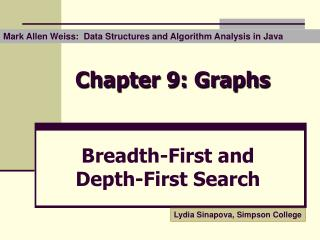DownloadDownload PresentationChapter 9: Graphs

# Chapter 9: Graphs

Télécharger la présentation## Chapter 9: Graphs

- - - - - - - - - - - - - - - - - - - - - - - - - - - E N D - - - - - - - - - - - - - - - - - - - - - - - - - - -
##### Presentation Transcript

1. Mark Allen Weiss: Data Structures and Algorithm Analysis in Java Chapter 9: Graphs Breadth-First and Depth-First Search Lydia Sinapova, Simpson College

2. Breadth-First and Depth-First Search • BFS Basic Algorithm • BFS Complexity • DFS Algorithm • DFS Implementation • Relation between BFS and DFS

3. BFS – Basic Idea Given a graph with N vertices and a selected vertex A:  for(i = 1; there are unvisited vertices ; i++) Visit all unvisited vertices at distance i (i is the length of the shortest path between A and currently processed vertices) Queue-based implementation

4. BFS – Algorithm BFS algorithm 1. Store source vertex S in a queue and mark as processed 2. while queue is not empty Read vertex vfrom the queue for all neighbors w: If w is not processed Mark as processed Append in the queue Record the parent of w to be v(necessary only if we need the shortest path tree)

5. Breadth-first traversal: 1, 2, 3, 4, 6, 5 1: starting node 2, 3, 4 : adjacent to 1 (at distance 1 from node 1) 6 : unvisited adjacent to node 2. 5 : unvisited, adjacent to node 3 Example 1 Adjacency lists 1: 2, 3, 4 2: 1, 3, 6 3: 1, 2, 4, 5, 6 4: 1, 3, 5 5: 3, 4 6: 2, 3 4 2 3 5 The order depends on the order of the nodes in the adjacency lists 6

6. Shortest Path Tree Consider the distance table: The table defines the shortest path tree, rooted at A.

7. BFS – Complexity Step 1 : read a node from the queue O(V) times. Step 2 : examine all neighbors, i.e. we examine all edges of the currently read node. Not oriented graph: 2*E edges to examine Hence the complexity of BFS isO(V + 2*E)

8. Depth-First Search Proceduredfs(s) mark all vertices in the graph as not reached invoke scan(s) Procedurescan(s) mark and visit s for each neighbor w of s if the neighbor is not reached invoke scan(w)

9. Depth-First Search with Stack Initialization: mark all vertices as unvisited, visit(s) while the stack is not empty: pop (v,w) if w is not visited add (v,w) to tree T visit(w) Procedure visit(v) mark v as visited for each edge (v,w) push (v,w) in the stack

10. Recursive DFS DepthFirst(Vertex v) visit(v); for each neighborw of v if (w is not visited) add edge (v,w) to tree T DepthFirst(w) Visit(v) mark v as visited

11. Example Depth first traversal: 1, 2, 6, 3, 5, 4 the particular order is dependent on the order of nodes in the adjacency lists 1 4 Adjacency lists 1: 2, 3, 4 2: 6, 3, 1 3: 1, 2, 6, 5, 4 4: 1, 3, 5 5: 3, 4 6: 2, 3 2 3 5 6

12. bfs(G) list L = empty tree T = empty choose a starting vertex x visit(x) while(L nonempty) remove edge (v,w) from beginning of L if w not visited add (v,w) to T visit(w) BFS and DFS dfs(G) list L = empty tree T = empty choose a starting vertex x visit(x) while(L nonempty) remove edge (v,w) from end of L if w not visited add (v,w) to T visit(w) Visit ( vertex v) mark v as visited for each edge (v,w) add edge (v,w) to end of L

13. Applications of DFS • Trees: preorder traversal • Graphs: • Connectivity • Biconnectivity – articulation points • Euler graphs